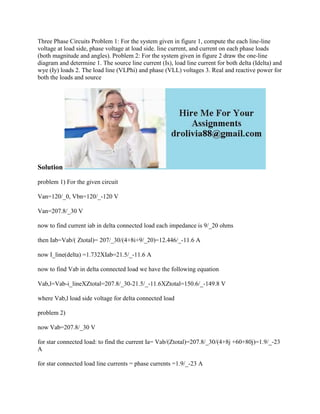Ce diaporama a bien été signalé.
Le téléchargement de votre SlideShare est en cours. ×

# Three Phase Circuits Problem 1- For the system given in figure 1- comp.docx

Publicité
Publicité
Publicité
Publicité
Publicité
Publicité
Publicité
Publicité
Publicité
Publicité
PublicitéChargement dans…3
×

## Consultez-les par la suite

1 sur 2 Publicité

# Three Phase Circuits Problem 1- For the system given in figure 1- comp.docx

Three Phase Circuits Problem 1: For the system given in figure 1, compute the each line-line voltage at load side, phase voltage at load side. line current, and current on each phase loads (both magnitude and angles). Problem 2: For the system given in figure 2 draw the one-line diagram and determine 1. The source line current (Is), load line current for both delta (Idelta) and wye (Iy) loads 2. The load line (VLPhi) and phase (VLL) voltages 3. Real and reactive power for both the loads and source
Solution
problem 1) For the given circuit
Van=120/_0, Vbn=120/_-120 V
Van=207.8/_30 V
now to find current iab in delta connected load each impedance is 9/_20 ohms
then Iab=Vab/( Ztotal)= 207/_30/(4+8i+9/_20)=12.446/_-11.6 A
now I_line(delta) =1.732XIab=21.5/_-11.6 A
now to find Vab in delta connected load we have the following equation
Vab,l=Vab-i_lineXZtotal=207.8/_30-21.5/_-11.6XZtotal=150.6/_-149.8 V
problem 2)
now Vab=207.8/_30 V
for star connected load: to find the current Ia= Vab/(Ztotal)=207.8/_30/(4+8j +60+80j)=1.9/_-23 A
for star connected load line currents = phase currents =1.9/_-23 A
Vph, Y= Vab/1.732=120/_30 V
for delta connected load: to find the cuurent Iab= Vab/(Ztotal)= 207.8/_30/(4+8j+60-9j)=3.24/_30 A
now line current I_line= 1.732X3.24/_30=5.62/_30A
1) Is=I_line (of delta)=5.62/_30A, Is=I_line(of star)=1.9/_-23 A
.

Three Phase Circuits Problem 1: For the system given in figure 1, compute the each line-line voltage at load side, phase voltage at load side. line current, and current on each phase loads (both magnitude and angles). Problem 2: For the system given in figure 2 draw the one-line diagram and determine 1. The source line current (Is), load line current for both delta (Idelta) and wye (Iy) loads 2. The load line (VLPhi) and phase (VLL) voltages 3. Real and reactive power for both the loads and source
Solution
problem 1) For the given circuit
Van=120/_0, Vbn=120/_-120 V
Van=207.8/_30 V
now to find current iab in delta connected load each impedance is 9/_20 ohms
then Iab=Vab/( Ztotal)= 207/_30/(4+8i+9/_20)=12.446/_-11.6 A
now I_line(delta) =1.732XIab=21.5/_-11.6 A
now to find Vab in delta connected load we have the following equation
Vab,l=Vab-i_lineXZtotal=207.8/_30-21.5/_-11.6XZtotal=150.6/_-149.8 V
problem 2)
now Vab=207.8/_30 V
for star connected load: to find the current Ia= Vab/(Ztotal)=207.8/_30/(4+8j +60+80j)=1.9/_-23 A
for star connected load line currents = phase currents =1.9/_-23 A
Vph, Y= Vab/1.732=120/_30 V
for delta connected load: to find the cuurent Iab= Vab/(Ztotal)= 207.8/_30/(4+8j+60-9j)=3.24/_30 A
now line current I_line= 1.732X3.24/_30=5.62/_30A
1) Is=I_line (of delta)=5.62/_30A, Is=I_line(of star)=1.9/_-23 A